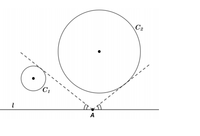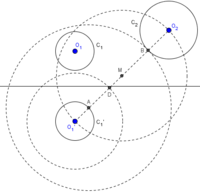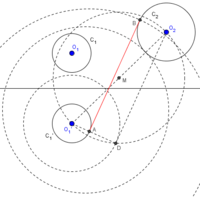# Reflexion problem. Can't Solve

#### CarlosP

##### New member
A line 1 and two circles C1 and C2 are given,
disjuncts of l, situated in the same semiplane bounded by l. Tell how to construct with a ruler and measure a point A in l such that there are two lines running through A, one tangent to C1 and the other to C2, which make the same angle with l.

https://prnt.sc/os2w1t (this is the picture of the problem)

I think i need reflect one line tangent to C2 , so the angle will be the same . But don't know which line i need choose for that lines have tangent to C1 and the other to C2 which make the same angle with l. Can someone help me ? Thanks

#### Dr.Peterson

##### Elite Member
Here is your picture, more easily accessed:I would reflect one circle across the line, so the problem becomes constructing a common tangent to two circles.

I believe there will be four solutions, corresponding to the four common tangents of a pair of circles.

But I'm not sure what methods you are allowed to use, as the problem doesn't specify the usual compass and straightedge, but rather measuring with a ruler.

#### CarlosP

##### New member
Ok you already helped me. Yes i understand i need reflect one circle across the line , but how do i construct a common tangent to this two circles? Thank you for the help#### tkhunny

##### Moderator
Staff member
Ok you already helped me. Yes i understand i need reflect one circle across the line , but how do i construct a common tangent to this two circles? Thank you for the helpPonder on the radius of each circle and how it might meet the "common" tangent. What is the minimum distance such a meeting point? What is the relationship between this minimum distance radius and the common tangent?

#### CarlosP

##### New member
Ponder on the radius of each circle and how it might meet the "common" tangent. What is the minimum distance such a meeting point? What is the relationship between this minimum distance radius and the common tangent?
Sorry but didn't understand your question. This picture is a sample, the teacher wants a answer that works for all examples. So i just need describe what i need to do.

#### Dr.Peterson

##### Elite Member
As I asked before, please tell us what kind of construction you are expected to do. You said to use a "ruler", which implies you can measure distances, rather than just draw lines. You didn't mention using a compass. Are you supposed to calculate certain distances and measure them out? Or is this a typical compass and straightedge construction, and you just didn't say so?

Also, what have you tried? If you can show us how you do the reflection, that might help us see what you are allowed to do, and what parts you understand, which we can extend to the problem of constructing a tangent. (I assumed the latter might be something you have seen before.)

#### CarlosP

##### New member
We can't measure distances with ruler, we just can use ruler to do lines or something like that , and compass to do circles or using to reflect.
The way i do the reflection of C1 across the line l is like that :
1) i put the compass in C1 and take the radius r1
2) we choose 2 points in l such that 1 are in the left of center C1(point A) and the other on the right of center C1 (point B)
3) consider the circumference of center A and radius AO1 (O1 is the center of C1) and the same in point B
4) the intersection of that two circumference we have O1 and point D
5) the reflection of C1 across the line l have center in D
6) now we draw with compass the circumference C1' with radius r1 and then we have the reflection of C1 across the line l

#### tkhunny

##### Moderator
Staff member
Sorry but didn't understand your question...
That's why I started with the word "ponder".

#### CarlosP

##### New member
That's why I started with the word "ponder".
now you understand what we can use and not ?
thanks

#### Dr.Peterson

##### Elite Member
Yes, you are doing a compass and straightedge construction.

Now it's time to ponder ...

The way I start a question like this (how to construct a common tangent to two given circles) is to sketch what the final result will look like, including any lines (such as radii to the points of tangency, and a line joining the centers of the circles) that will help describe the situation.

Do that. You should see a right-angled trapezoid (you may call it a trapezium). If you could make a line parallel to the base (which is your line of tangency), you would be well on your way to constructing the latter.

Don't expect to see an answer immediately; like any interesting problem, you will have to take several steps, each of which may be a smaller construction in itself that you will have learned, or you may even have to invent these as well.

#### CarlosP

##### New member
Thank you for the help, can you see if that answer is right ?

1) we reflect C1 across the line 1 ( like i said before )
2) we will find the common tangency of C1' and C2 that are under radii O1' and O2
3) to find that common tangency we do the line O1'O2 ( O1' center of C1' and O2 center of C2 ), then we find mediatrix of the line O1'O2 and let M be that point.
4) now we put the compass in M and draw a circle with radii O1'M. Then we put the compass in O1' and draw a circle with radii r1'+r2. The intersection point under the line O1'O2 will be the point D.
5) now we to the line O1'D and when that line cross C1' we call that point A
6) Then with compass we mark the lenght DO2 and we put the compass in A and mark the point B in C2 with that lenght ( that point B will be under O1'O2 )
7) The intersection of AB in the line l will be the point we are looking for.

#### Dr.Peterson

##### Elite Member
I'd never seen the word "mediatrix" in this context before, but apparently it is a term for the perpendicular bisector of a segment. You are apparently using the term to mean the midpoint of the segment.

Did you mean |r1'-r2| rather than r1'+r2? If so, then your construction is essentially this one (which I found by searching for my phrase "construct a common tangent to two given circles", and which agrees with what I would have done, while also explaining the reasoning nicely).

Good work.

#### CarlosP

##### New member
Yes , we in portugal use the term "mediatriz" that is mediatrix in english. don't know if that term in english is correct, but mediatrix is like you said ( midpoint of the segment ).
When i said r1' + r2 i want say the sum of radiis , we use that to construct the common tangent. I used that method before. Is that method wrong and should i use |r1'-r2| rather then r1' + r2?
And the rest of my answer to find the point in l such that there are two lines running through that point, one tangent to C1 and the other to C2, which make the same angle with l , is right?Thank you for the help

#### Dr.Peterson

##### Elite Member
Actually, I hadn't tried carrying out your construction in detail; I see that it is much more different than I though, so I was wrong.

But I have apparently misinterpreted something, because I can't get past step 5 or so. (There are a few words, like "under", that I am not sure of, and step 5 is missing a word.) Here is my drawing as far as I got:Can you explain the reasoning behind your construction?

By the way, here is the one place I saw "mediatrix" defined for geometry in English; the proper word for what you want is "midpoint".

#### CarlosP

##### New member
5) now we draw the line O1'D and when that line cross C1' we call that point A

Basically , in my thoughts to solve that problem i think i need reflect C1 across the line l so when i find a common tangency between C1' and C2 we have the same angle ( for example , imagine that tangency point in C1'is A and the tangency point in C2 is B , and when that line cross l is the point D. Then the angle AD do the same angle that BD in the line l , this is a theorem of geometry between 2 vertically lines ). So thats why i try solve like this .But i'm not sure is that right.

#### Dr.Peterson

##### Elite Member
I notice now that I didn't use the circle centered at M; I suspect that in step 4, D should be the intersection of the two circles just drawn. In fact, now I see that "under" (which from previous lines I had taken as your way of saying "on") means "toward the bottom of the drawing". We don't usually describe things that way, so I didn't read it that way.

Here is my new drawing.Yes, this is equivalent to the construction I showed you, except that you are constructing one of the two internal common tangents rather than an external common tangent. Good work.

#### CarlosP

##### New member
Then my answer is right ? Really thank you for helping me and point me the right direction to solve the problemsorry for my bad english

#### Dr.Peterson

##### Elite Member
Yes, your answer is correct. The link I provided for the construction may have some additional information you would find helpful to understand things more fully, but you did well.

Your English is far better than my Portuguese (or even my French, which I actually studied long ago).

•CarlosP

#### CarlosP

##### New member
Thank you for your kind words , and for provide me some help without showing me the answer•Dr.Peterson